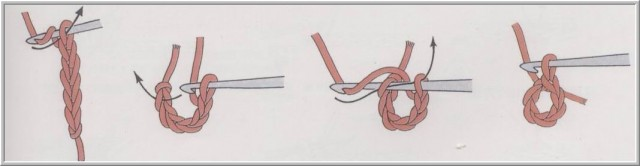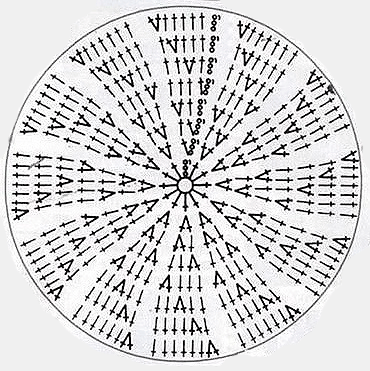# How to Knit in a circle hookUsing the hook and the thread can be various geometric shapes vyvyazyvayut. One of the simplest, of which is a circle. Knit in a circle hook is not difficult, and in some cases even easier, than a simple rectangular cloth. By learning vyvyazyvayut this geometrical figure, then you can safely explore knitting techniques napkins, headgear, as well as many other interesting articles.

## Where to start knitting circle hook

Usually, start knitting circle hook, you can use two basic and most common ways:

• Having a ring of thread,
• Linking chain ring, related several stitches.

In that case, if you want to knit a tight circle, without the formation of holes in it in the middle of canvas, the best option would be to start knitting circle from one loop. This is done as follows::

•Start of thread are such, to form a circular loop, thus it is necessary not to forget to leave the tip, equal to about two centimeters.
• The loop created by introducing the hook, We grab a thread and stretch it over. Loop at the same time it is not necessary to tighten.
• Then re-enter the hook in the loop from a thread, and then pull the thread through her work, and then passed through the loop, and, vyvyazannuyu first, making, in this way, the connecting bar. It is important when making this action lightly hold the thumb and middle finger the place of crossing thread ring, to work was not disbanded.
• At the next stage in the ring of the thread provyazyvaetsya required number of columns without nakida.
• At the end of a series of work necessary to pull the left end of the thread, gently tighten the middle of a circle, so that the central hole turned out quite unnoticeable.

Knit ring of the chain is also very simple. For this:

• Vyvyazyvayut chain of the required number of air loops, eg, of three.
• Threading the hook in the first loop of the chain, pull the thread through the work.
• Whereupon the loop is pulled through the last loop, pre-connected chain, as if vyvyazyvaya polustolbik.
• Then, in the resulting ring provyazyvaem bars without sc, so many, as necessary to specifically of knitting. And knit columns as possible in the ring itself, and it loops, wherein the pre-allocating an equal amount for each.## How to learn how to crochet circle

Crochet circle, you can use any columns, and using stitches, the main ones are polustolbiki, as well as columns with and without sc . In order to get a smooth result, knitting circle, you need to know a few secrets of its performance:

1. Any knitting rows in a circle begins and ends exactly the same. At the beginning of the lifting loops have to do, and at the end vyvyazyvayut polustolbiki from the last column of the previous series in the first, connecting its end and the beginning.
2. In order to avoid confusion in the knitting and easily find the beginning of each row, primary loop number is necessary to mark with a special pin or thread segment than the base fabric color.
3. It is important to evenly distribute in a circle number vyvyazyvayut columns.
4. If the circle is without columns sc, knitting starts from the three columns without sc, then in the next row of the number of doubles, that is, in the end it turns out 6 petelek in a row. Further knitting is divided into six fractions and each subsequent number is the addition of a uniform 1 loop for each part, that is, each number should increase every time on the 6 loops. On will help knit circle crochet scheme is presented below5. Knitting circle columns with sc, initial round should consist of 6 loops or bars, without sc, in the case of knitting the beginning of the air loop. In the second row the number of loops is doubled for 12 loops in a row and the possibility of dividing the circle in the future 12 parts. Then a twelfth of each added one loop, so that for every row of the addition was found to be 12 eyelet. Preview of the knitting process further possible thanks to the following scheme:6. If the circle you want to associate columns with a large number sc, the addition in each row must be large, than in the previous case, 4 loop. In this way, for columns with two sc every row increases by 16 loops, three sc on 20 and so on.
7. When knitting circle sc polustolbikami with each row will need to be increased by 8 loops. For this initial round is knitted from 4 loops, and the second is increased to eight. Then, as in the previous embodiments, the circle is divided into equal parts, but this time with a total of eight. It is at the beginning of each of these additional parts and provyazyvaetsya polustolbik with nakida. This is done as follows:, in first loop klinushka provyazyvaetsya two polustolbika with sc, and in the following to the end 1/8 part one.

After you have mastered the basic techniques, crochet circle can safely take on more complex openwork patterns, or make circles using thread of different colors.